# Number Systems

Explore the world of number systems and their properties by going through its various aspects. Find answers to questions like what is a number system, how they are formed, the difference between binary, octal, decimal, and hexadecimal numbers, easy ways to solve problems on them, and commonly used properties of numbers from different systems.

Become a champ by playing around with interactive simulations and solving interesting questions on number systems.

A number system is a system of representing numbers. It is also called “the system of numeration”.It defines a set of values to represent a quantity.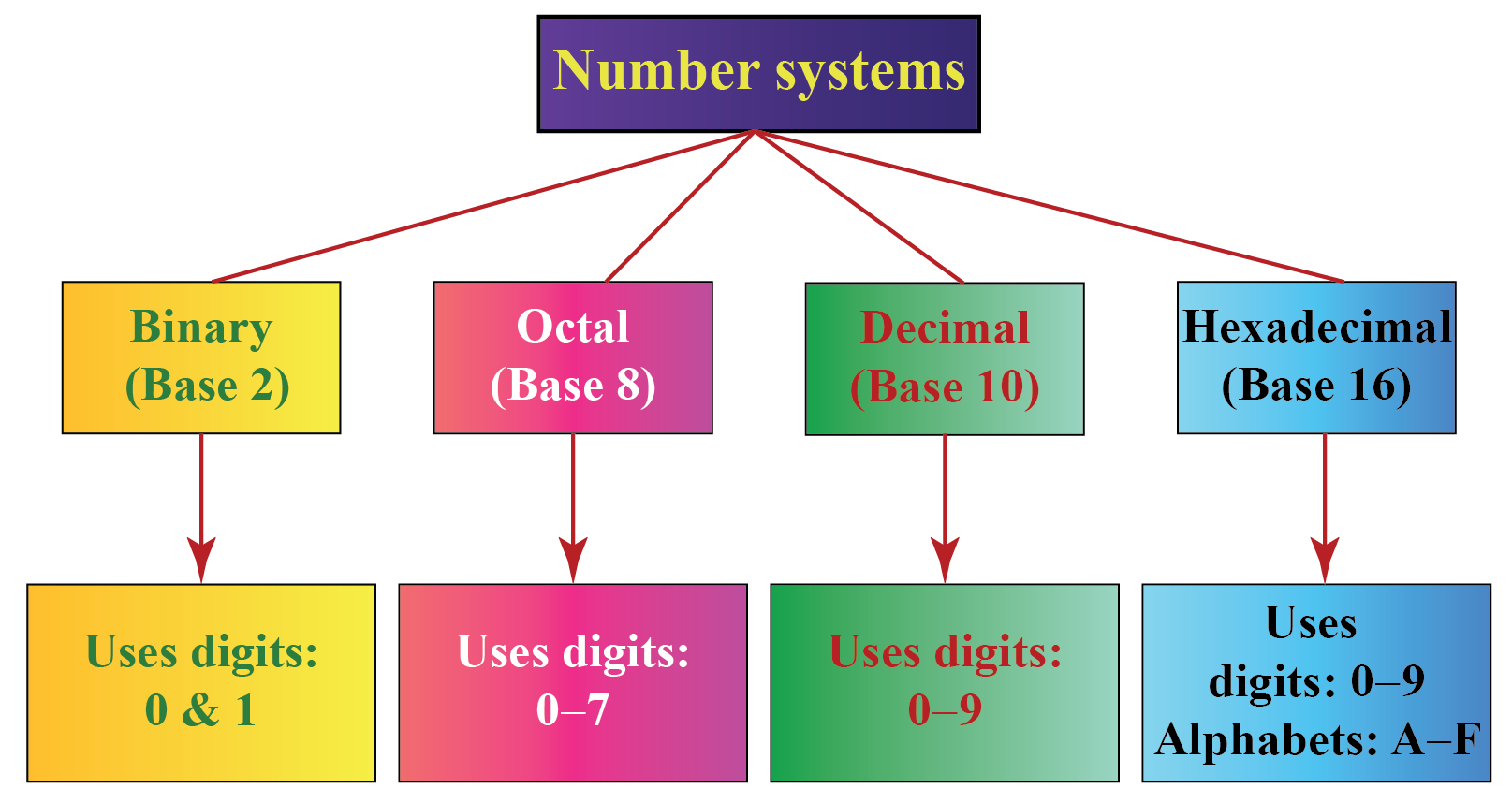1 What Does Number System Mean? 2 Learn These Along with Number System! 3 Solved Examples on Number System 4 Interactive Questions on Number System

## What Does Number System Mean?

### Types of Number Systems in Maths

We have different types of number systems in Maths. But there are $$4$$ main types of number systems:

1. Binary number system (Base - $$2$$)
2. Octal number system  (Base - $$8$$)
3. Decimal number system  (Base - $$10$$)
4. Hexadecimal number system  (Base - $$16$$)

We will study each of these systems one by one in detail.

### Binary Number System

• The binary number system uses only two digits: $$0$$ and $$1$$.

• Thus the numbers in this system have a base $$2$$.

• $$0$$ and $$1$$ are called bits.

• $$8$$ bits together make a byte.

• The data in computers is stored in terms of bits and bytes.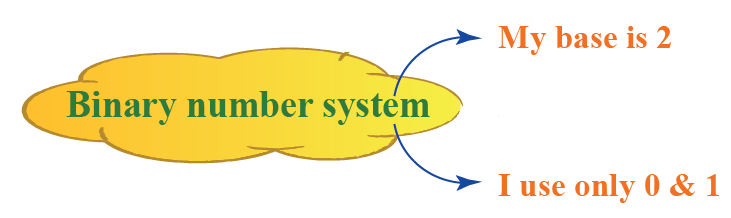Examples:

$$10001_2, 111101_2, 1010101_2$$ are some examples of numbers in the binary number system.

### Octal Number System

• The octal number system uses eight digits: $$0,1,2,3,4,5,6$$ and $$7$$.

• Thus the numbers in this system have a base $$8$$.

• The advantage of this system is it has less number of digits when compared to several other systems and hence there would be fewer computational errors.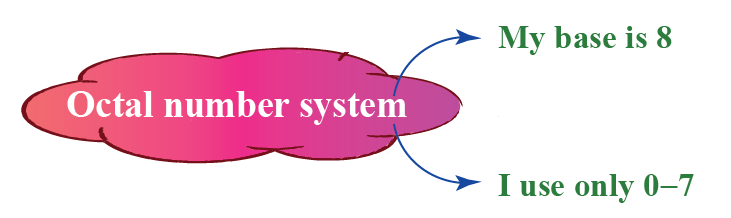Examples:

$$35_{8}, 923_{8}, 141_{8}$$ are some examples of numbers in the octal number system.

### Decimal Number System

• The decimal number system uses ten digits: $$0,1,2,3,4,5,6,7,8$$ and $$9$$.

• Thus the numbers in this system have a base $$10$$.

• If we see any number without any base, it means that its base is $$10$$.

• This is the system that we generally use to represent the numbers in real life.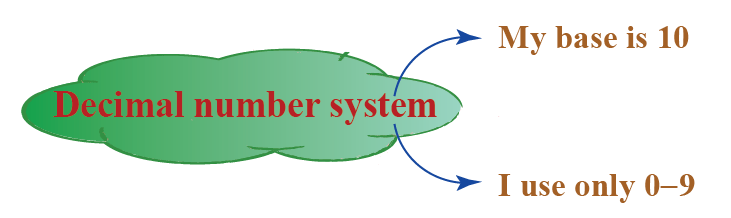Example 4327  in the decimal number system.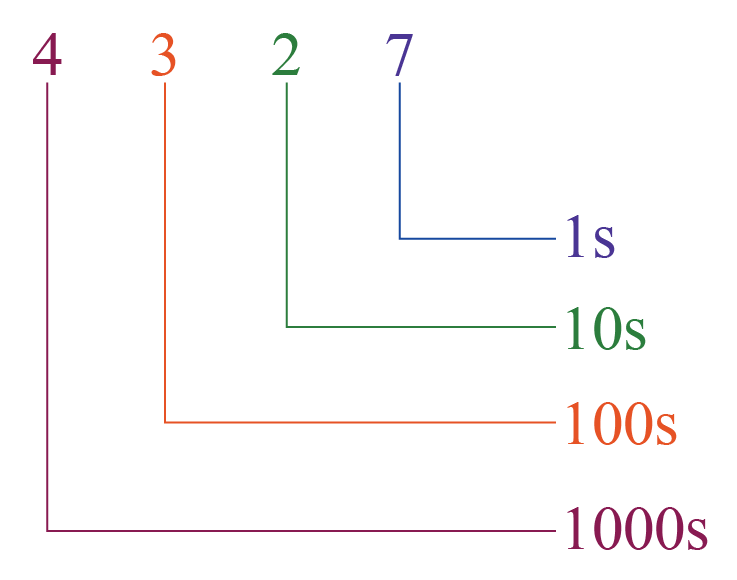Examples:

$$723_{10}, 32_{10}, 4257_{10}$$ are some examples of numbers in the decimal number system.

• The hexadecimal number system uses sixteen digits/alphabets: The digits $$0,1,2,3,4,5,6,7,8, 9$$ and the alphabets $$A,B,C,D, E, F$$.

• Thus the numbers in this system have a base $$16$$.

• Here, $$A-F$$ of the hexadecimal system means the numbers $$10-15$$ of the decimal number system respectively.

• This system is used in computers to reduce the large-sized strings of the binary system.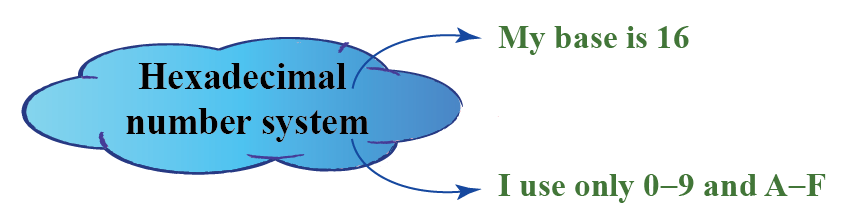Examples:

$$7B3_{16}, 6F_{16}, 4B2A_{16}$$ are some examples of numbers in the hexadecimal number system.

### Difference Between Decimal and Binary Number Systems

The differences between the decimal and binary number systems are as follows:

Decimal Number System Binary Number System
It uses $$10$$ digits:$$0,1,2,3,4,5,6,7,8$$ and $$9$$ It uses $$2$$ digits: $$0$$ and $$1$$.
Its base is $$10$$ and it doesn’t need to be written always to show that the number is in the decimal number system. Its base is $$2$$ and it has to be written to show that the number is in the binary number system.
It is the most general system used in daily life. It is the system used by the computers to store and process the data.

### Number Systems Chart

The information about number systems is summarised in the following chart:### Number Systems Conversions

A number can be converted from one number system to another number system.

We will see how to do this.

### Binary / Octal / Hexadecimal System to Decimal System

To convert a number from binary/octal/hexadecimal system to the decimal system, we use the following steps.

The steps are shown by an example of a number in the binary system.

Example:

Convert $$100111_2$$ into the decimal system.

Solution:

Step 1: Identify the base of the given number.

Here, the base of $$100111_2$$ is $$2$$.

Step 2: Multiply each digit of the given number, starting from the rightmost digit, with the exponents of the base.

The exponents should start with $$0$$ and increase by $$1$$ every time as we move from right to left.

Since the base here is $$2$$, we multiply the digits of the given number by $$2^0, 2^1, 2^2,...$$ from right to left.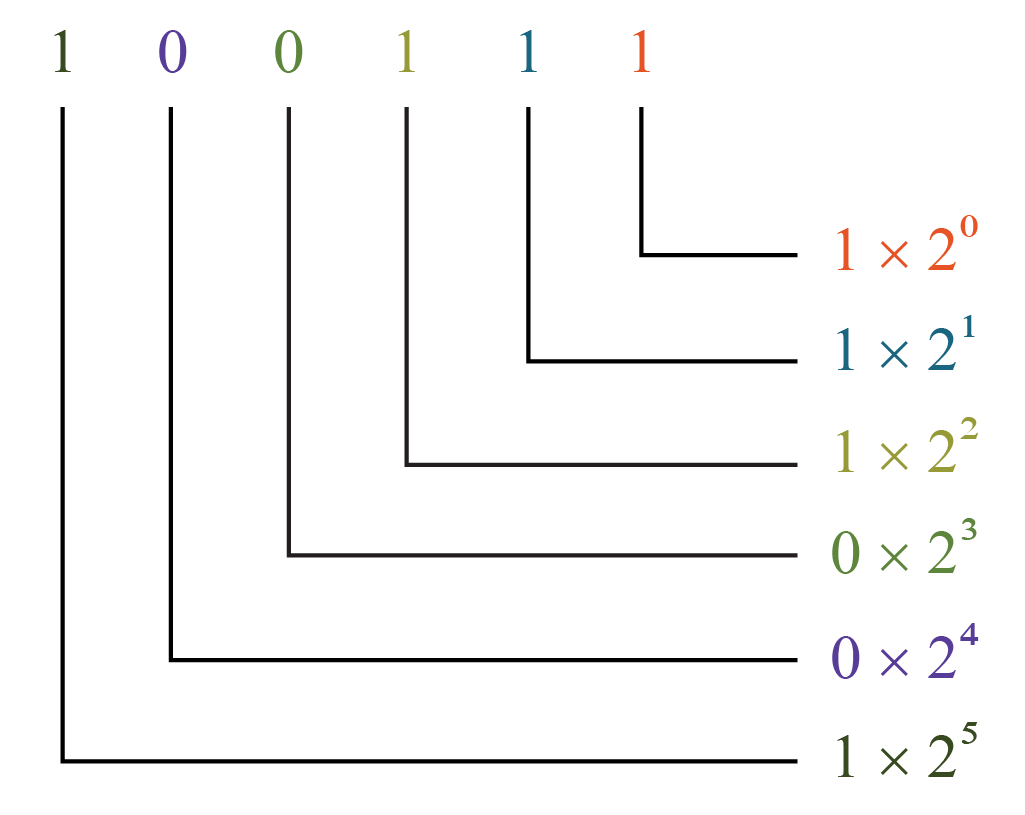Step 3: We just simplify each of the above products and add them.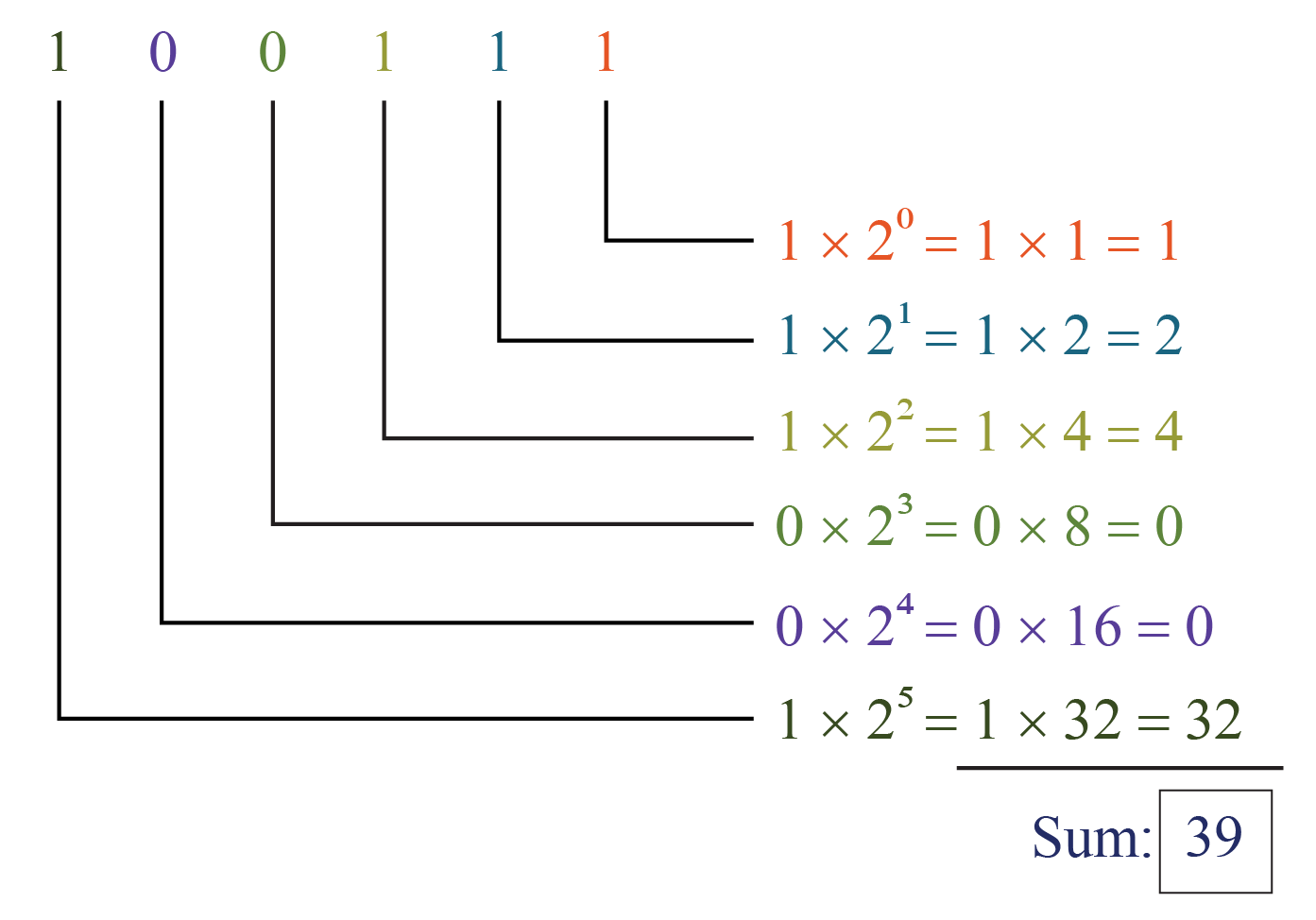Here, the sum is the equivalent number in the decimal number system of the given number.

Or, we can use the following steps to make this process simplified.

\begin{align} 100111 &= 1 \times 2^5 + 0 \times 2^4 + 0 \times 2^3+ 1 \times 2^2 + 1 \times 2^1 + 1 \times 2^0 \\[0.3cm] &= 1 \times 32 + 0 \times 16 + 0 \times 8 + 1 \times 4 + 1 \times 2 + 1 \times 1 \\[0.3cm] &= 32 +0+0+4+2+1\\[0.3cm] &= 39 \end{align}
Thus,

 $$\therefore 100111_2 = 39_{10}$$

### Decimal to Binary / Octal / Hexadecimal System

To convert a number from decimal system to binary / octal / hexadecimal system, we use the following steps.

The steps are shown on how to convert a number from the decimal system to the octal system.

Example:

Convert $$4320_{10}$$ into the octal system.

Solution:

Step 1: Identify the base of the required number.

Since we have to convert the given number into the octal system, the base of the required number is $$8$$.

Step 2: Divide the given number by the base of the required number and note down the quotient and the remainder in the “quotient-remainder” form.

Repeat this process (dividing the quotient again by the base) until we get the quotient to be less than the base.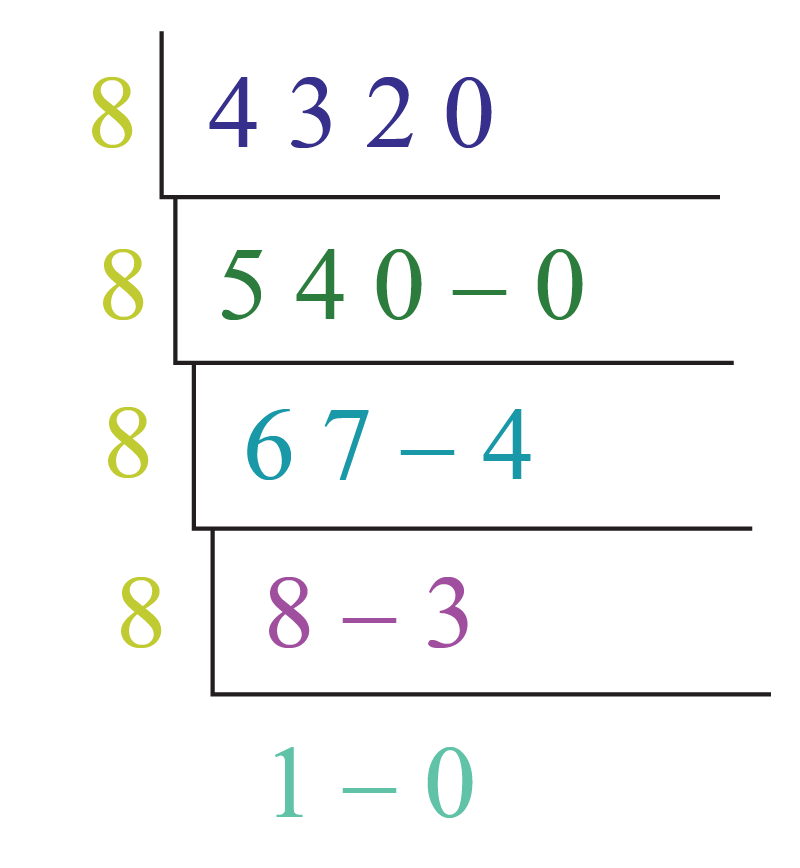Step 3: The given number in the octal number system is obtained just by reading all the remainders and the last quotient from bottom to top.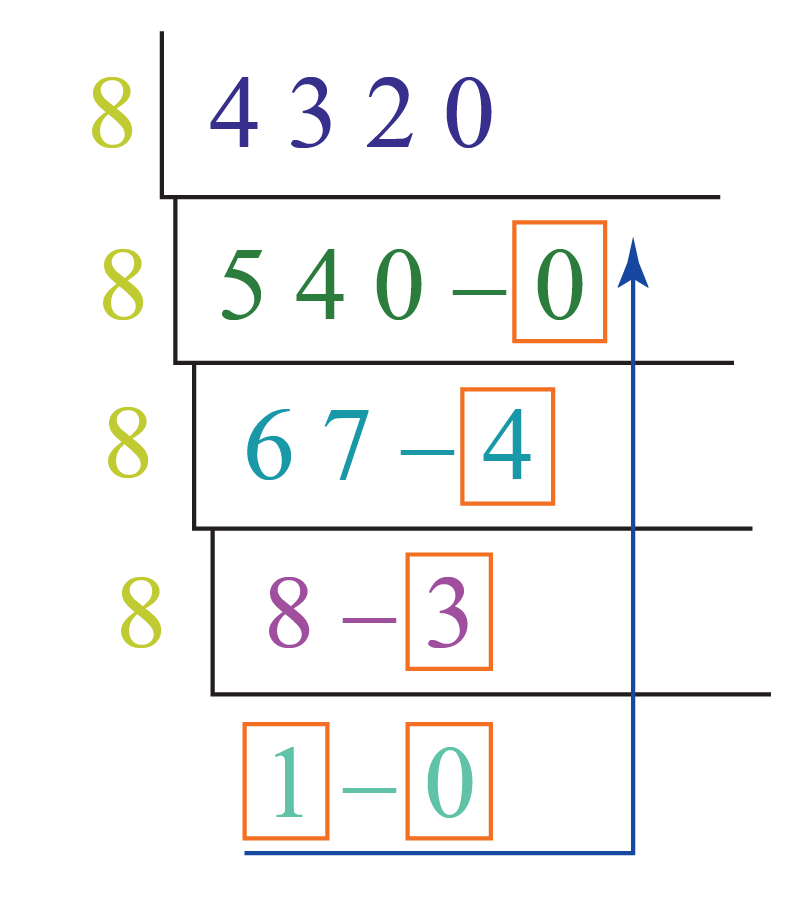Thus,

 $$\therefore 4320_{10} = 10340_{8}$$

### One System to Another System

To convert a number from one of the binary/octal/hexadecimal systems to one of the other systems, we first convert it into the decimal system, and then we convert it to the required systems by using the above-mentioned processes.

Example:

Convert $$1010111100_2$$ to the hexadecimal system.

Solution:

Step 1: Convert this number to the decimal number system as explained in the above process.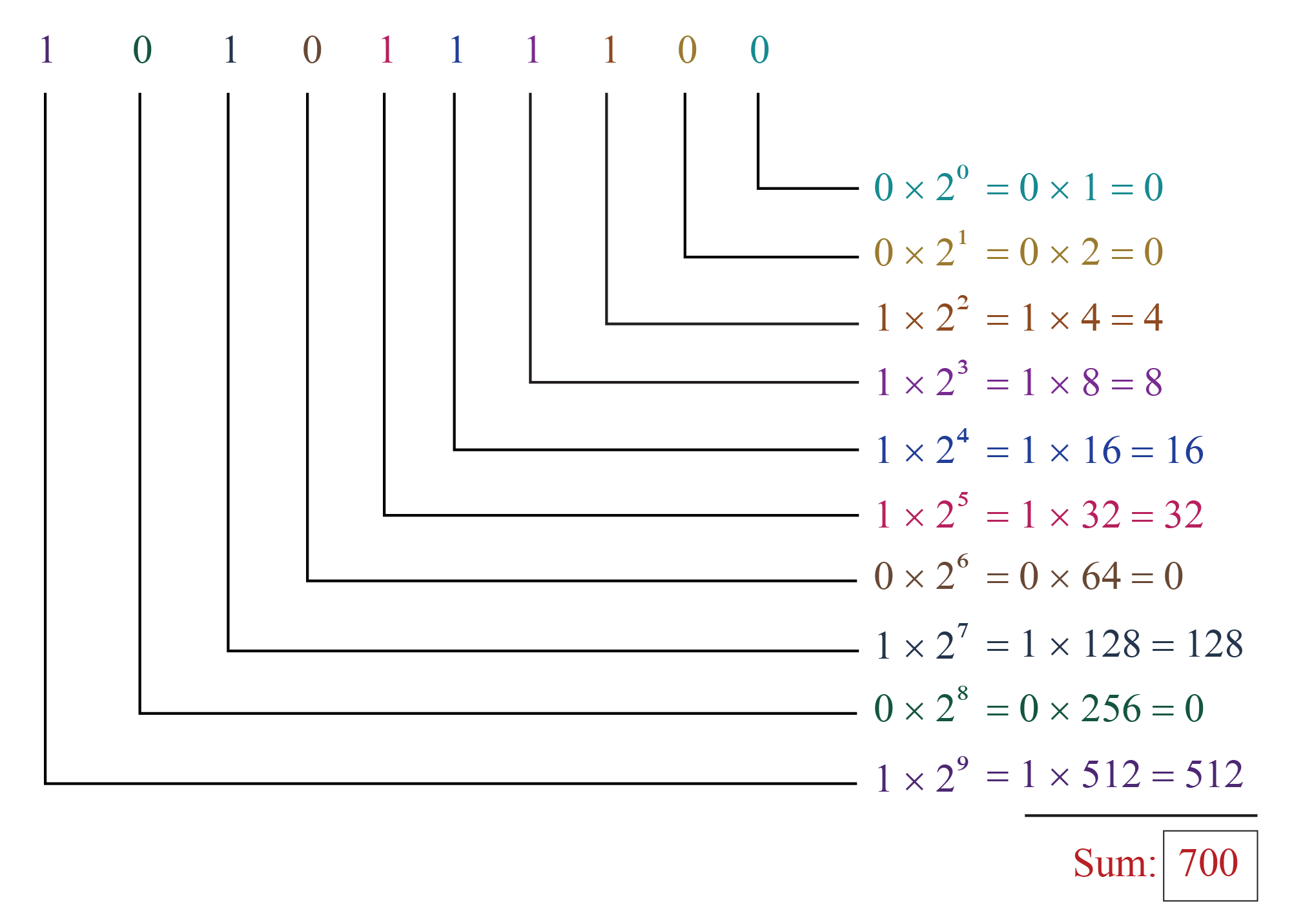Thus,

$1010111100_2 = 700_{10} \rightarrow (1)$

Step 2: Convert the above number (which is in the decimal system), into the required number system.

Here, we have to convert $$700_{10}$$ into the hexadecimal system using the above-mentioned process.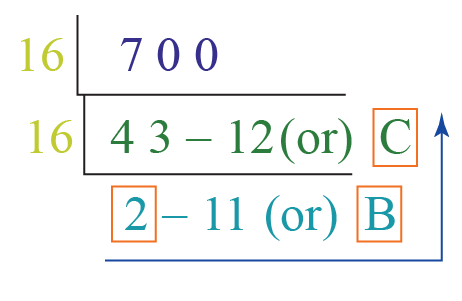Thus,

$700_{10} = 2BC_{16} \rightarrow (2)$

From the equations (1) and (2),

 $$1010111100_2 = 2BC_{16}$$Important Notes
1. To convert a number from binary/octal/hexadecimal system to decimal system:

Multiply each digit of the given number, starting from the rightmost digit, with the exponents of the base, where the exponents start from $$0$$ and increase by $$1$$ every time. Then just add the above products.

2. To convert a number from decimal system to binary/octal/hexadecimal system:

Divide the given number by the base of the required number and note down the quotient and the remainder in the “quotient-remainder” form repetitively until we get the quotient to be less than the base. The given number in the decimal number system is obtained just by reading all the remainders and the last quotient from bottom to top.### Number Systems Conversion Calculator

You can enter a number in one of the desired systems and the following calculator will show the equivalent number in the other systems.

## Learn These Along with Number System!

Here are a few more lessons related to the number system.

These topics will not only help you master the concept for the number system, but also other topics related to it.

 Check out other related topics. Click on any of the short lessons you want to explore. Numbers in Words Indian Numeral System Pre-number Math What are Numbers Indian Place Value Chart Natural Numbers Numbers up to 2 digits Numbers up to 3 digits Numbers up to 4 digits Numbers up to 5 digits Numbers up to 6 digits

## Solved Problems on Number System

 Example 1

Convert $$300_{10}$$ into the binary system (base- $$2$$).

Solution:

$$300_{10}$$ is in the decimal system.

We divide $$300$$ by $$2$$ and note down the quotient and the remainder.

We will repeat this process for every quotient until we get a quotient which is less than $$2$$.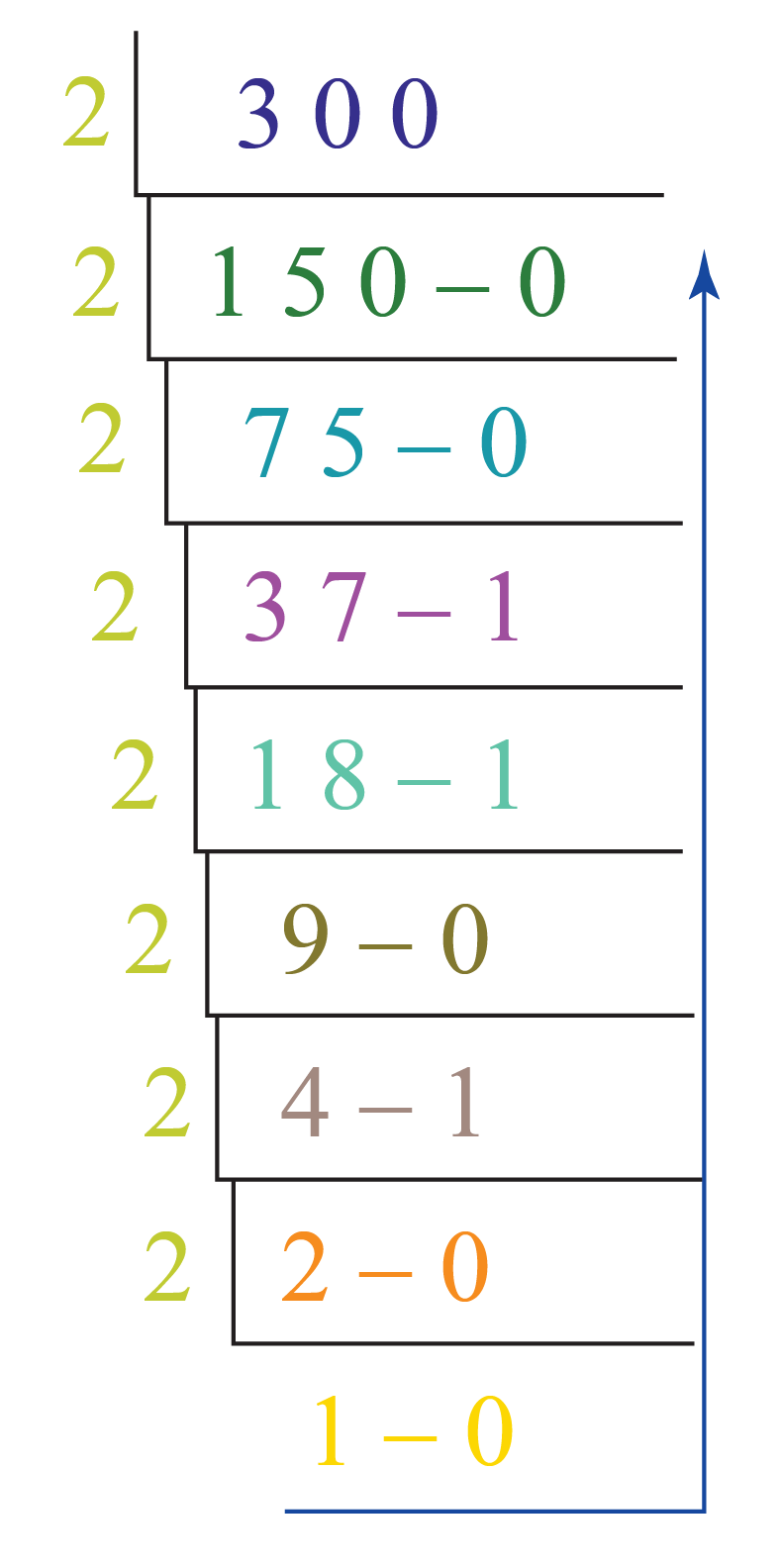The equivalent number in the binary system is obtained by reading all the remainders and just the last quotient from bottom to top as shown above.

Thus,

 $$300_{10} = 100101100_2$$
 Example 2

Convert $$5BC_{16}$$ into the decimal system.

Solution:

$$5BC_{16}$$ is in the hexadecimal system.

We know that $$B=11$$ and $$C= 12$$ in the hexadecimal system.

So we get the equivalent number in the decimal system using the following process: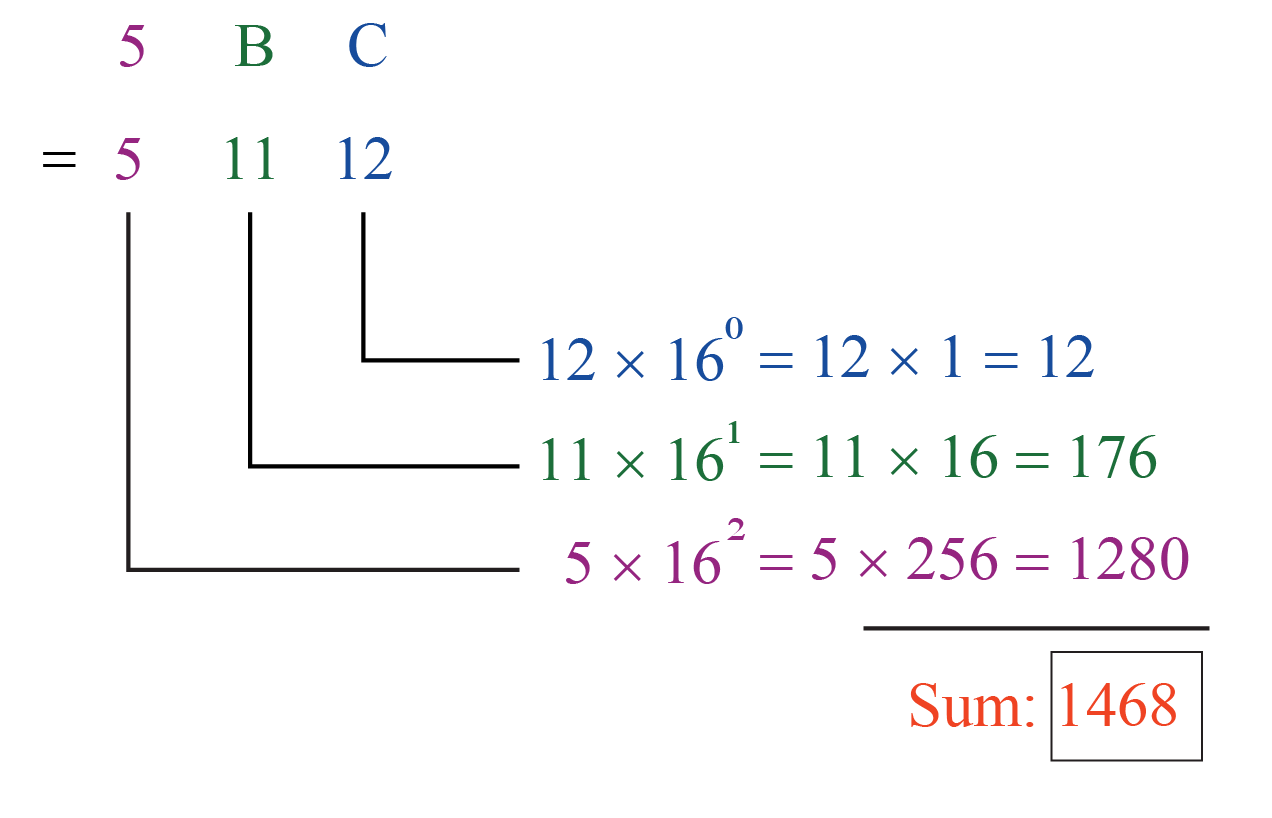Thus,

 $$5BC_{16}= 1468_{10}$$
 Example 3

Convert $$144_8$$ into the hexadecimal system.

Solution:

The base of $$144_8$$ is $$8$$.

First, we will convert this number into the decimal system as follows: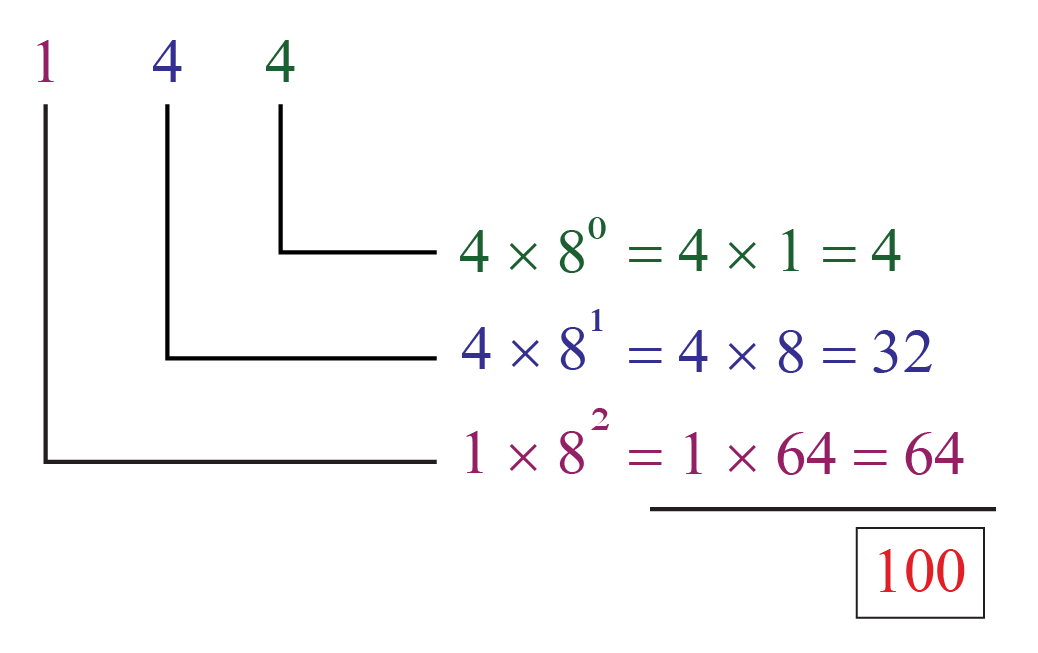Thus,

$144_8= 100_{10} \rightarrow (1)$

Now we will convert this into the hexadecimal system as follows: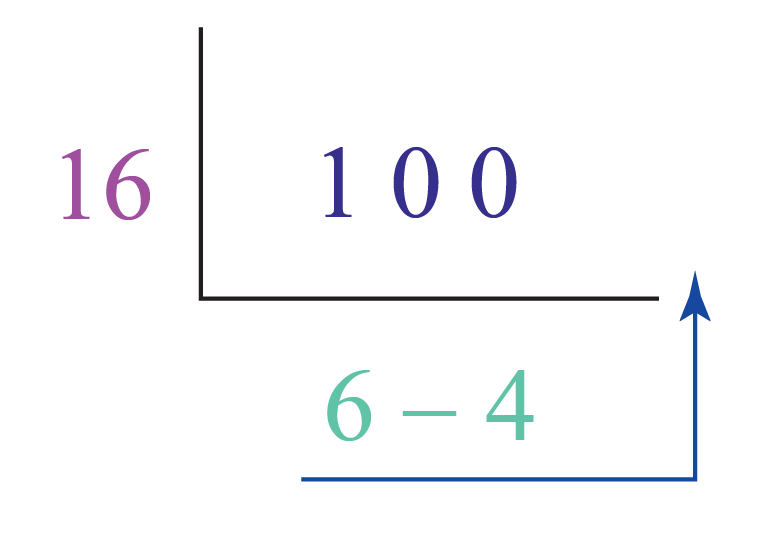Thus,

$100_{10} = 64_{16} \rightarrow (2)$

From the equations (1) and (2), we can conclude that:

 $$144_8= 64_{16}$$Challenging Questions
1. Convert $$111000_2$$ into the decimal system.
2. Convert $$347_8$$ into the decimal system.
3. Convert $$3AF_{16}$$ into the binary system.
4. Convert $$300_{10}$$ into the binary system.

## Interactive Questions on Number System

Here are a few problems related to number systems.

## Summary

The magic of math lies in the amazing concepts that it is built upon. At Cuemath, we explore and interact with these concepts in a fun way!

The math journey around number systems starts with what a student already knows, and goes on to creatively crafting a fresh concept in the young minds. Done in a way that not only it is relatable and easy to grasp, but also will stay with them forever.

The best part, this isn’t the end. With a universe built around number systems at Cuemath, one can take their math journey forward with solved examples, practice questions, quizzes, worksheets, practice papers, and so much more. Again, all of it, exclusively around number systems.

## 1. What are the 4 types of number systems?

There are $$4$$ main types of number systems:

• Binary number system (Base - $$2$$)
• Octal number system  (Base - $$8$$)
• Decimal number system  (Base - $$10$$)
• Hexadecimal number system  (Base - $$16$$)

## 2. What is a number system with examples?

A number system is a system of representing the numbers. Some examples of numbers in different number systems are $10010_2, 234_8, 428_{10}, 4BA_{16}$

## 3. What are the rules of a number system?

To convert a number from binary/octal/hexadecimal system to decimal system:

1. Multiply each digit of the given number, starting from the rightmost digit, with the exponents of the base.
2. The exponents should start with $$0$$ and increase by $$1$$ every time as we move from right to left.
3. We just simplify each of the above products and add them.

To convert a number from decimal system to binary/octal/hexadecimal system:

1. Divide the given number by the base of the required number and note down the quotient and the remainder in the “quotient-remainder” form.
2. Repeat this process (dividing the quotient again by the base) until we get the quotient to be less than the base.
3. The given number in the decimal number system is obtained just by reading all the remainders and the last quotient from bottom to top.

To convert a number from one of the binary/octal/hexadecimal systems to one of the other systems:

1. We first convert it into the decimal system.
2. Then we convert it to the required system.

## 4. Why are the number systems used?

There are different purposes of each number system, such as:

• The binary number system is used to store the data in computers.
• The advantage of the octal number system is it has less number of digits when compared to several other systems and hence, there would be fewer computational errors.
• The decimal number system is the system that we use in daily life.
• The hexadecimal number system is used in computers to reduce the large-sized strings of the binary system.

More Important Topics
Numbers
Algebra
Geometry
Measurement
Money
Data
Trigonometry
Calculus